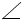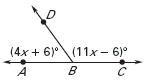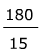# Supplementary Angles

The definition of supplementary is two angles whose sum is 180° are supplementary. The angles can be either adjacent (share a common side and a common vertex and are side-by-side) or non-adjacent.

Example 1. Given m 1 = 45° and m 2=135° determine if the two angles are supplementary.

45° + 135° = 180° therefore the angles are supplementary.

We will use the following facts to help us determine if two angles are supplementary.

• A linear pair is two angles that are adjacent and form a line.

• The angle measure of a line is 180°

• If two angles form a linear pair then they are supplementary.

Example 2: the angles form a line (linear pair) therefore they are supplementaryExample 3: the angles can be non-adjacent as long as their sum is 180°110°+ 70° = 180° The sum is 180° therefore they are supplementary.

Example 4:1 and2 form a linear pair so m1 + m2 = 180° therefore the angles are supplementary.How many other linear pairs can you see in the diagram?m2 + m3 = 180°     m3 + m4 = 180°     m1 + m4 = 180°

Remember that linear pairs are supplementary and that 2 intersecting lines will form 4 pairs of supplementary angles.

Geometry & Algebra: find the value of x the find the mABD and mDBC.Write an equation (4x +6) ° + (11x - 6)°= 180°

Identify like terms (4x +6) ° + (11x - 6)°= 180°

Combine like terms 4x + 11x +6 - 6 = 180°

15x + 0 = 180°

The zero is unnecessary=Divide both sides by 15

x = 12

mABD = 4x + 6 = 4(12)+6 = 54°

mDBC = 11x - 6 = 11(12) -6 = 126°